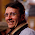## Saturday, September 11, 2010

### Chocolates and roses

You have 21 chocolates and 91 roses, how many bouquets can you make without discarding neither a rose nor a chocolate. All the bouquets must have the same number of chocolates and roses for you.

1.2.7 bouquet's of 13 roses and 3 chocolates each
rowan

3.yep 7 (13, 3). correct.

4.The formal solution should be:

91 # 21 (mod x) (where # must be read congruency)

so that:
91-21 = k x => 70/k=x

now we sould find a k, divisor of 70 such as:
91 mod x = 0 and 21 mod x = 0
k=10 e x=7 are out solution